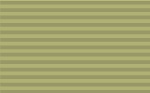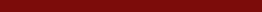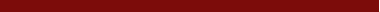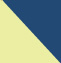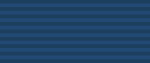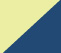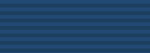Manifolds Topological Manifolds (Lee Chapter 1) Smooth Manifolds (Lee Chapter 1) Smooth Functions (Lee 2) Tangent Spaces and Linearlization Tangent spaces (Lee Chapter 3) Linearization and the local behavior of functions (Lee first section of Chapter 4) Submanifolds Immersions and Submanifolds (Lee Chapter 5 and last part of 4) Submersions (Lee Chapter 5 and last part of 4) Whitney embedding theorem (Lee Chapter 6) Bundles (Lee Chapter 10) Fiber bundles Vector bundles Vector Fields Vector fields (Lee Chapter 8) Flows (Lee Chapter 9) Lie derivaitves (Lee Chapter 9) Approximation and Stability Approximation of continuous functions (Lee Chapter 6, Section 4) Normal bundles and tubular neighborhoods (Lee Chapter 6, Section 4) Approximation of continuous functions II (Lee Chapter 6, Section 4) Homotopy and Stability (Guillemin and Pollack Chapter 1, Section 6) Transversality Genericity of transversality (Lee Chapter 6, Section 5) 1-manifolds and applications (Guillemin and Pollack Chapter 2, Section 2) Mod 2 intersection theory (Guillemin and Pollack Chapter 2, Section 4) Cotangent bundles and 1-forms Linear algebra (Lee Chapter 11) Cotangent bundles (Lee Chapter 11) Integration (Lee Chapter 11) Tensors Linear algebra (Lee Chapter 12) Tensor fields (Lee Chapter 12) Forms Linear algebra (Lee Chapter 14) k-forms (Lee Chapter 14) Exterior derivative Lie derivative (Lee Chapter 14) Integration Orientation (Lee Chapter 15) Integration (Lee Chapter 16) Stokes theorem (Lee Chapter 16) DeRham cohomology (Lee Chapter 17) Products and Poincare Duality Oriented intersection and degree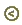home